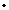Discussion Meeting
ORGANIZERS
Prahladh Harsha (TIFR, India), Ramprasad Saptharishi (TIFR, India) and Srikanth Srinivasan (IIT-Bombay, India)
DATE & TIME
25 March 2019 to 29 March 2019
VENUE

Algebraic complexity aims at understanding the computational aspects of algebraic objects such as multivariate polynomials, tensors etc. The primary focus in this field has been the study of multivariate polynomials, and its hardness based on the number of addition/multiplication operations required to compute it (i.e. via algebraic circuits'). This is a quest to answer the VP vs VNP'' question, an algebraic analogue of the classical `P vs NP'' question and is a fundamental open problem in algebraic complexity.

These questions about multivariate polynomial broadly fall in one of the following categories:

1. Lower bounds: Can we find explicit polynomials that cannot be computed by small algebraic circuits?
2. Polynomial Identity Testing: Given an algebraic circuit C, can we test efficiently if the circuit C is computing the zero polynomial? (Or equivalently, can we test if two circuits C_1 and C_2 are computing the same polynomial?)
3. Reconstruction: Given blackbox access to a circuit C, can we query evaluations of this blackbox to reconstruct a small circuit for C?

These above problems, albeit very different at first glance, are intimately connected to each other. Over the last 7-8 years, there has been tremendous progress in understanding the above questions for natural subclasses of circuits, the deep relationships between these problems, and also some barrier results to give clarity on what types of techniques are promising approaches to the general problems. This five day workshop would have a series of about 25-30 lectures by various researchers in the field comprising of some of the recent advances in the field and also some tutorials aimed at giving students a gentle introduction to the area.

Accommodation for outstation/non-local participants will be provided at our on campus ICTS guest house.

wact2019ictsresin